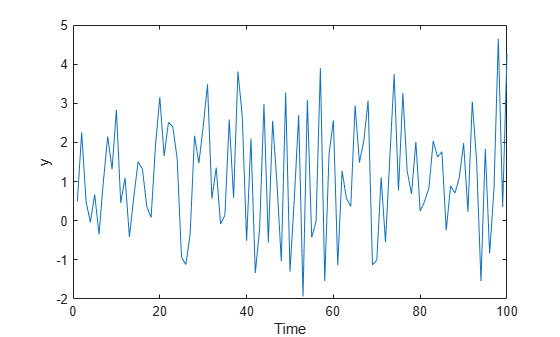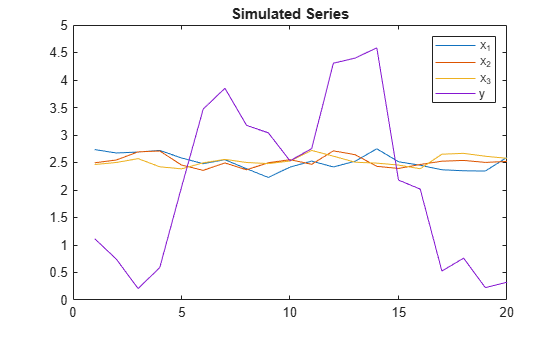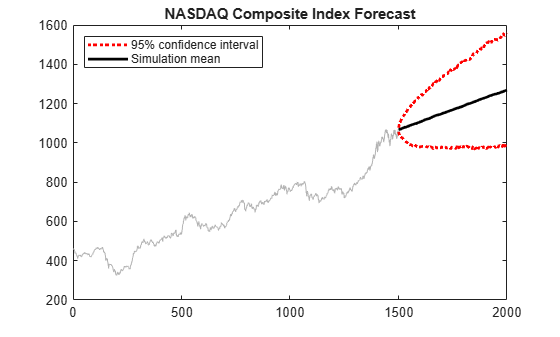# simulate

Monte Carlo simulation of ARIMA or ARIMAX models

## Syntax

``````[Y,E] = simulate(Mdl,numObs)``````
``[Y,E] = simulate(Mdl,numObs,Name=Value)``
``````[Y,E,V] = simulate(___)``````

## Description

example

``````[Y,E] = simulate(Mdl,numObs)``` simulates length `numObs` sample response and innovations paths, `Y` and `E`, respectively, from the ARIMA model `Mdl`. The responses can include the effects of seasonality.```

example

````[Y,E] = simulate(Mdl,numObs,Name=Value)` specifies additional options using one or more name-value arguments. For example, `simulate(Mdl,10,NumPaths=1000,Y0=y0)` simulates `1000` sample paths of length `10` from the ARIMA model `Mdl`, and uses the observations in `y0` as a presample to initialize each generated path.```

example

``````[Y,E,V] = simulate(___)``` also simulates paths of conditional variances `V` for a composite conditional mean and variance model (for example, an ARIMA and GARCH composite model) using any of the input argument combinations in the previous syntaxes.```

## Examples

collapse all

Consider the ARIMA(4,1,1) model

`$\left(1-0.75{L}^{4}\right)\left(1-L\right){y}_{t}=2+\left(1+0.1L\right){\epsilon }_{t},$`

where ${\epsilon }_{\mathit{t}}$ is a Gaussian innovations series with a mean of 0 and a variance of 1.

Create the ARIMA(4,1,1) model.

```Mdl = arima('AR',-0.75,'ARLags',4,'MA',0.1,... 'Constant',2,'Variance',1)```
```Mdl = arima with properties: Description: "ARIMA(4,0,1) Model (Gaussian Distribution)" Distribution: Name = "Gaussian" P: 4 D: 0 Q: 1 Constant: 2 AR: {-0.75} at lag  SAR: {} MA: {0.1} at lag  SMA: {} Seasonality: 0 Beta: [1×0] Variance: 1 ```

`Mdl` is a fully specified `arima` object representing the ARIMA(4,1,1) model.

Simulate a 100-period random response path from the ARIMA(4,1,1) model.

```rng(1) % For reproducibility y = simulate(Mdl,100);```

`y` is a 100-by-1 vector containing the random response path.

Plot the simulated path.

```plot(y) ylabel('y') xlabel('Period')```Simulate three predictor series and a response series.

Specify and simulate a path of length 20 for each of the three predictor series modeled by

`$\left(1-0.2L\right){x}_{it}=2+\left(1+0.5L-0.3{L}^{2}\right){\eta }_{it},$`

where ${\eta }_{it}$ follows a Gaussian distribution with mean 0 and variance 0.01, and $i$ = {1,2,3}.

```[MdlX1,MdlX2,MdlX3] = deal(arima('AR',0.2,'MA',... {0.5,-0.3},'Constant',2,'Variance',0.01)); rng(4); % For reproducibility simX1 = simulate(MdlX1,20); simX2 = simulate(MdlX2,20); simX3 = simulate(MdlX3,20); SimX = [simX1 simX2 simX3];```

Specify and simulate a path of length 20 for the response series modeled by

`$\left(1-0.05L+0.02{L}^{2}-0.01{L}^{3}\right)\left(1-L{\right)}^{1}{y}_{t}=0.05+{x}_{t}^{\prime }\left[\begin{array}{c}0.5\\ -0.03\\ -0.7\end{array}\right]+\left(1+0.04L+0.01{L}^{2}\right){\epsilon }_{t},$`

where ${\epsilon }_{t}$ follows a Gaussian distribution with mean 0 and variance 1.

```MdlY = arima('AR',{0.05 -0.02 0.01},'MA',... {0.04,0.01},'D',1,'Constant',0.5,'Variance',1,... 'Beta',[0.5 -0.03 -0.7]); simY = simulate(MdlY,20,'X',SimX);```

Plot the series together.

```figure plot([SimX simY]) title('Simulated Series') legend('{X_1}','{X_2}','{X_3}','Y')```Forecast the daily NASDAQ Composite Index using Monte Carlo simulations.

Load the NASDAQ data included with the toolbox. Extract the first 1500 observations for fitting.

```load Data_EquityIdx nasdaq = DataTable.NASDAQ(1:1500); n = length(nasdaq);```

Specify, and then fit an ARIMA(1,1,1) model.

```NasdaqModel = arima(1,1,1); NasdaqFit = estimate(NasdaqModel,nasdaq);```
``` ARIMA(1,1,1) Model (Gaussian Distribution): Value StandardError TStatistic PValue _________ _____________ __________ __________ Constant 0.43031 0.18555 2.3191 0.020392 AR{1} -0.074391 0.081985 -0.90737 0.36421 MA{1} 0.31126 0.077266 4.0284 5.6158e-05 Variance 27.826 0.63625 43.735 0 ```

Simulate 1000 paths with 500 observations each. Use the observed data as presample data.

```rng default; Y = simulate(NasdaqFit,500,'NumPaths',1000,'Y0',nasdaq);```

Plot the simulation mean forecast and approximate 95% forecast intervals.

```lower = prctile(Y,2.5,2); upper = prctile(Y,97.5,2); mn = mean(Y,2); figure plot(nasdaq,'Color',[.7,.7,.7]) hold on h1 = plot(n+1:n+500,lower,'r:','LineWidth',2); plot(n+1:n+500,upper,'r:','LineWidth',2) h2 = plot(n+1:n+500,mn,'k','LineWidth',2); legend([h1 h2],'95% Interval','Simulation Mean',... 'Location','NorthWest') title('NASDAQ Composite Index Forecast') hold off```Simulate response and innovation paths from a multiplicative seasonal model.

Specify the model

`$\left(1-L\right)\left(1-{L}^{12}\right){y}_{t}=\left(1-0.5L\right)\left(1+0.3{L}^{12}\right){\epsilon }_{t},$`

where ${\epsilon }_{t}$ follows a Gaussian distribution with mean 0 and variance 0.1.

```Mdl = arima('MA',-0.5,'SMA',0.3,... 'SMALags',12,'D',1,'Seasonality',12,... 'Variance',0.1,'Constant',0);```

Simulate 500 paths with 100 observations each.

```rng default % For reproducibility [Y,E] = simulate(Mdl,100,'NumPaths',500); figure subplot(2,1,1); plot(Y) title('Simulated Response') subplot(2,1,2); plot(E) title('Simulated Innovations')```Plot the 2.5th, 50th (median), and 97.5th percentiles of the simulated response paths.

```lower = prctile(Y,2.5,2); middle = median(Y,2); upper = prctile(Y,97.5,2); figure plot(1:100,lower,'r:',1:100,middle,'k',... 1:100,upper,'r:') legend('95% Interval','Median')```Compute statistics across the second dimension (across paths) to summarize the sample paths.

Plot a histogram of the simulated paths at time 100.

```figure histogram(Y(100,:),10) title('Response Distribution at Time 100')```## Input Arguments

collapse all

Fully specified ARIMA model, specified as an `arima` model object created by `arima` or `estimate`.

The properties of `Mdl` cannot contain `NaN` values.

Sample path length, specified as a positive integer. That is, the number of random observations to generate per output path. `Y`, `E`, and `V` have `numObs` rows.

Data Types: `double`

### Name-Value Arguments

Specify optional pairs of arguments as `Name1=Value1,...,NameN=ValueN`, where `Name` is the argument name and `Value` is the corresponding value. Name-value arguments must appear after other arguments, but the order of the pairs does not matter.

Before R2021a, use commas to separate each name and value, and enclose `Name` in quotes.

Example: `simulate(Mdl,10,NumPaths=1000,Y0=y0)` simulates `1000` sample paths of length `10` from the ARIMA model `Mdl`, and uses the observations in `y0` as a presample to initialize each generated path.

Number of independent sample paths to generate, specified as a positive integer. The output arguments `Y`, `E`, and `V` have `NumPaths` columns.

Example: `NumPaths=1000`

Data Types: `double`

Presample response data used as initial values for the model, specified as a numeric column vector or a numeric matrix.

Each row of `Y0` corresponds to a period in the presample. The following conditions apply:

• The last row contains the latest presample responses.

• To initialize the AR components, `Y0` must have at least `Mdl.P` rows.

• If `Y0` has more rows than is required to initialize the model, `simulate` uses only the latest required rows.

Each column of `Y0` corresponds to a separate, independent presample path. The following conditions apply:

• If `Y0` is a column vector, `simulate` applies it to each path. In this case, the AR components and conditional variance model of all paths in `Y` derive from common initial responses.

• If `Y0` is a matrix, `simulate` applies `Y0(:,j)` to initialize path `j`. `Y0` must have at least `NumPaths` columns; `simulate` uses only the first `NumPaths` columns of `Y0`.

By default, `simulate` sets any necessary presample observations by using one of the following methods:

• For a model with a stationary AR process and without a regression component, `simulate` sets all presample responses to the unconditional mean of the model.

• For a model that represents a nonstationary process or that contains a regression component, `simulate` sets all necessary presample responses to zero.

Data Types: `double`

Presample innovation data used to initialize either the moving average (MA) component of the ARIMA model or the conditional variance model, specified as a numeric column vector or a numeric matrix. `simulate` assumes the presample innovations have a mean of zero.

Each row of `E0` corresponds to a period in the presample. The following conditions apply:

• The last row contains the latest presample innovations.

• To initialize the MA components, `E0` must have at least `Mdl.Q` rows.

• If `Mdl.Variance` is a conditional variance model (for example, a `garch` model object), `E0` can require more rows than `Mdl.Q` to initialize the model.

• If `E0` has more rows than is required to initialize the model, `simulate` uses only the latest required rows.

Each column of `E0` corresponds to a separate, independent presample path. The following conditions apply:

• If `E0` is a column vector, `simulate` applies it to each simulated path. In this case, the MA components and conditional variance model of all paths in `Y` derive from the same initial innovations.

• If `E0` is a matrix, `simulate` applies `E0(:,j)` to initialize simulating path `j`. `E0` must have at least `NumPaths` columns; `simulate` uses only the first `NumPaths` columns of `E0`.

By default, `simulate` sets all necessary presample innovations to `0`.

Data Types: `double`

Presample conditional variance data used to initialize the conditional variance model, specified as a positive numeric column vector or a positive numeric matrix. If the conditional variance `Mdl.Variance` is constant, `simulate` ignores `V0`.

Each row of `V0` corresponds to a period in the presample. The following conditions apply:

• The last row contains the latest presample conditional variances.

• To initialize the conditional variance model, `V0` must have enough rows. For details, see the `simulate` function of conditional variance models.

• If `V0` has more rows than is required to initialize the conditional variance model, `simulate` uses only the latest required rows.

Each column of `V0` corresponds to a separate, independent presample path. The following conditions apply:

• If `V0` is a column vector, `simulate` applies it to each simulated path.

• If `V0` is a matrix, `simulate` applies `V0(:,j)` to initialize simulating path `j`. `V0` must have at least `NumPaths` columns; `simulate` uses only the first `NumPaths` columns of `V0`.

By default, `simulate` sets all necessary presample observations to the unconditional variance of the conditional variance process.

Data Types: `double`

Exogenous predictor data for the regression component in the model, specified as a `numObs`-by`numPreds` numeric matrix.

`numPreds` is the number of predictor variables (`numel(Mdl.Beta)`).

Each row of `X` corresponds to a period in the length `numObs` simulation sample (period for which `simulate` simulates observations; the period after the presample). The following conditions apply:

• The last row contains the latest predictor data.

• If the specified predictor data has more than `numObs` rows, `simulate` uses only the latest `numObs` rows.

• `simulate` does not use the regression component in the presample period.

Each column of `X` corresponds to a separate predictor variable.

`simulate` applies `X` to each simulated path; that is, `X` represents one path of observed predictors.

By default, `simulate` excludes the regression component, regardless of its presence in `Mdl`.

Data Types: `double`

Note

• `NaN`s in input data indicate missing values. `simulate` uses listwise deletion to delete all sampled times (rows) in the input data containing at least one missing value. Specifically, `simulate` performs these steps:

1. Synchronize, or merge, the presample data sets `E0`, `V0`, and `Y0` to create the set `Presample`.

2. Remove all rows from `Presample` and the predictor data `X` containing at least one `NaN`.

Listwise deletion applied to the in-sample data can reduce the sample size and create irregular time series.

• `simulate` assumes that you synchronize the predictor series such that the most recent observations occur simultaneously. The software also assumes that you synchronize the presample series similarly.

## Output Arguments

collapse all

Simulated response paths, returned as a length `numObs` numeric column vector or a `numObs`-by-`NumPaths` numeric matrix. `Y` represents the continuation of the presample responses in `Y0`.

Each row corresponds to a period in the simulated series; the simulated series has the periodicity of `Mdl`. Each column is a separate simulated path.

Simulated model innovations paths, returned as a length `numObs` numeric column vector or a `numObs`-by-`NumPaths` numeric matrix.

The dimensions of `E` correspond to the dimensions of `Y`.

Simulated conditional variance paths of the mean-zero innovations associated with `Y`, returned as a length `numObs` numeric column vector or a `numObs`-by-`NumPaths` numeric matrix.

The dimensions of `V` correspond to the dimensions of `Y`.

 Box, George E. P., Gwilym M. Jenkins, and Gregory C. Reinsel. Time Series Analysis: Forecasting and Control. 3rd ed. Englewood Cliffs, NJ: Prentice Hall, 1994.

 Enders, Walter. Applied Econometric Time Series. Hoboken, NJ: John Wiley & Sons, Inc., 1995.

 Hamilton, James D. Time Series Analysis. Princeton, NJ: Princeton University Press, 1994.